MORE IN Strength of Materials
SPPU Civil Engineering (Semester 3)
Strength of Materials
December 2014
Total marks: --
Total time: --
INSTRUCTIONS
(1) Assume appropriate data and state your reasons
(2) Marks are given to the right of every question
(3) Draw neat diagrams wherever necessary

Answer any one question from Q1 and Q2
1 (a) A steel bar 120 mm long, 40 mm ?40 mm in cross-section is subjected to tensile load 200 kN along longitudinal axis and tensile loads of 500 kN and 400 kN on lateral faces as shown in Fig. 1. Change in volume was observed to be 125mm3. Find the value of Poisson? ratio and Bulk Modulus. Take E = 200 Gpa.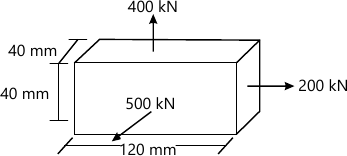6 M
1 (b) two Wooden Planks 150 mm × 50 mm each are connected to form a T section of a beam. A moment of 6.4 kN-m is applied around the horizontal neutral axis. Find the bending stresses at both the extreme fibers of cross-section (Fig. 2)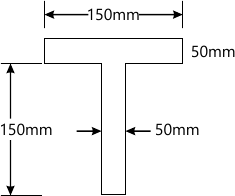6 M

2 (a) A composite member made of steel bar (60 mm dia. And 600 mm length) and aluminum bar (30 mm dia. and 300 mm length) is held between two supports as shown in Fig. 3. The composite member is stress free at a temperature 38°C. What will be the stress in the two materials, when temperature is 21°C. Assume supports are unyielding. Take ES=210 Gpa, EA= 74 Gpa and αs=11.7 ×10-6/°C, αA=23.4×10-6/°C.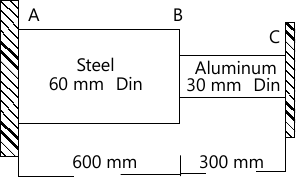6 M
2 (b) A beam of symmetrical I section is 200 mm × 400 mm in size. The thickness of flange is 20 mm and web is 15 mm. The beam is carrying maximum shear force 80 kN. Draw stress distribution diagram over the depth of section.
6 M

Answer any one question from Q3 and Q4
3 (a) A bronze tube of 80 mm outside diameter is fitted over and firmly attached to a steel shaft of 50 mm dia. The working shearing stresses are 65 MPa and 40 MPa for steel and bronze respectively. Calculate the power which can be transmitted by the compound shaft at 5 rpm. Take G = 85 GPa for steel and 45 GPa for bronze.
6 M
3 (b) A shaft of 100 mm diameter transmits 200 kW power at 200 rpm. If at a section bending moment is 5 kNm, then find the principal stress and maximum shear stress.
6 M

4 (a) A steel rod 80 mm in diameter is 5 m long. Find the maximum instantaneous stress induced when a pull of 160 kN is applied(i) Gradually (ii) Suddenly. Also find instantaneous elongation. comments on result. Take E=210 Gpa.
6 M
4 (b) Prove: $\dfrac {T} {J} = \dfrac {G \theta} {L} = \dfrac {\tau} {R}$ in case of torsion of circular shaft.
6 M

Answer any one question from Q5 and Q6
5 (a) A beam AB 10 m long and hinged at its ends is subjected to couples as shown in Fig. 4. Draw shear force diagram and bending moment diagram. Also determine the position of a point of contraflexure if any.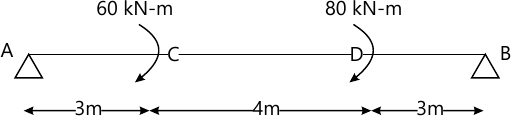7 M
5 (b) For the beam ABCD the shear force diagram as shown in Fig 5. Construct the bending moment diagram and load diagram from given shear force diagram. No couple is acting on the beam.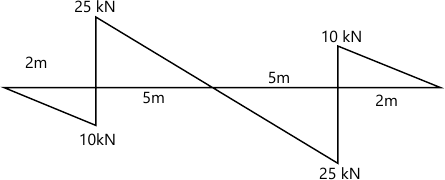6 M

6 (a) Draw shear force diagram and bending moment diagram for the beam as shown in Fig. 6. Indicate the numerical values at all important section. Find the position of contraflexure if any: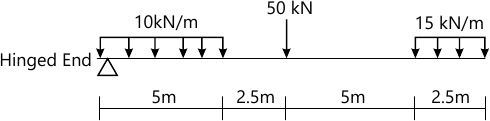7 M
6 (b) The diagram as shown in Fig. 7 is the shear force diagram for a beam which rests on two supports, one being at the left hand end.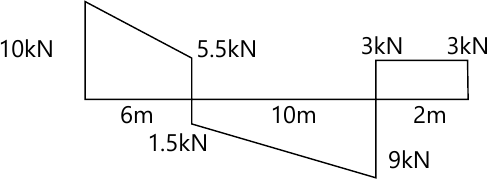6 M

Answer any one question from Q7and Q8
7 (a) Determine the crippling load when strut is used with both ends pinned or hinged.
6 M
7 (b) A short length of tube of 40 mm internal diameter and 50 mm external diameter failed in compression at a load of 240 kN. When 2 m length of the same tube was tested as a strut with fixed ends the load at failure was 158 kN. Assuming that 'sigma;C?in Rankine? formula is given by the first test, find the value of the constant ?alpha;?in same formula. What will be crippling load of this tube, if it is used as a strut 3 m long with one end fixed and the other end hinged ?
7 M

8 (a) A steel rod 5 m long and of 40 mm diameter is used as a column with one end fixed and other is free. Determine the crippling load by Euler? formula. Take E = 200 Gpa.
7 M
8 (b) A rectangular column section of size 400 mm × 200 mm is subjected to compressive eccentric load of 210 kN acting as shown in Fig. 8 at an eccentricity of 80 mm from longitudinal axis. Determine the maximum and minimum stresses set up in the section: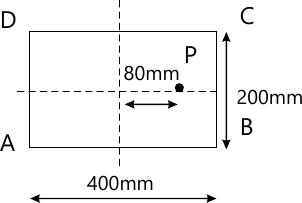7 M

More question papers from Strength of Materials